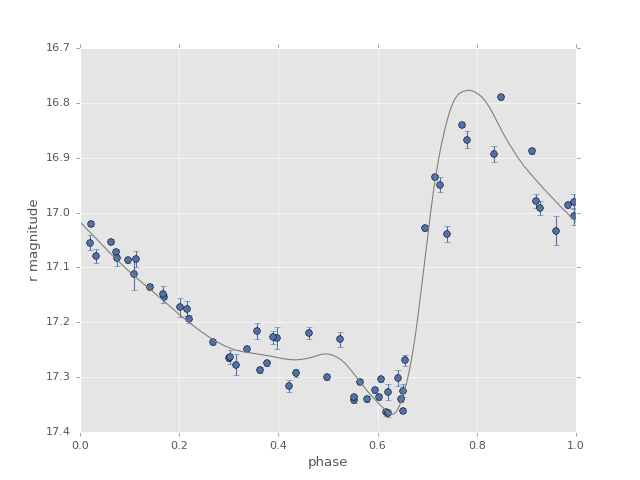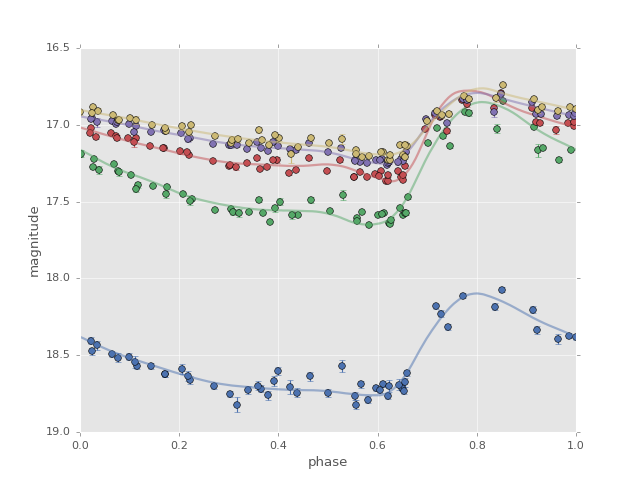# Template-based Period Fitting¶

Though it can be very slow in practice, a template-based fitting method is perhaps the best way to narrow-in on the period of astronomical objects, particularly if the templates fit the data well. The reason for the slow performance is that template models require a nonlinear optimization for each candidate period.

Note that while it is possible to use the period optimizer with the template method, we skip it here because it is very computationally intensive.

## Single Band Template Model¶

`gatspy` implements a template-based model using the Sesar 2010 RR Lyrae templates in the `RRLyraeTemplateModeler` class. We’ll demonstrate its use here, starting with fetching some RR Lyrae data:

```In : from gatspy import datasets, periodic

In : rrlyrae = datasets.fetch_rrlyrae()

In : lcid = rrlyrae.ids

In : t, mag, dmag, filts = rrlyrae.get_lightcurve(lcid)

In : mask = (filts == 'r')

```

Next we will define the template model and fit it to the data:

```In : model = periodic.RRLyraeTemplateModeler('r')

In : model.fit(t_r, mag_r, dmag_r);
```

With this model fit, we can now compute the light curve for any given period. This fit will compute the RMS residual around all available templates and use the one which provides the closest fit:

```In : period = rrlyrae.get_metadata(lcid)['P']

In : t_fit = np.linspace(0, period, 1000)

In : mag_fit = model.predict(t_fit, period=period)
```

Plotting the results, we see the best fit template at this phase:## Multiband Template Fitting¶

The multiband template model makes use of templates within each band, and fits each individually. This is implemented in `RRLyraeTemplateModelerMultiband`. The API for this modeler is similar to that discussed in the Multiband Lomb-Scargle Periodogram.

The following figure shows the template fits to a multiband lightcurve: